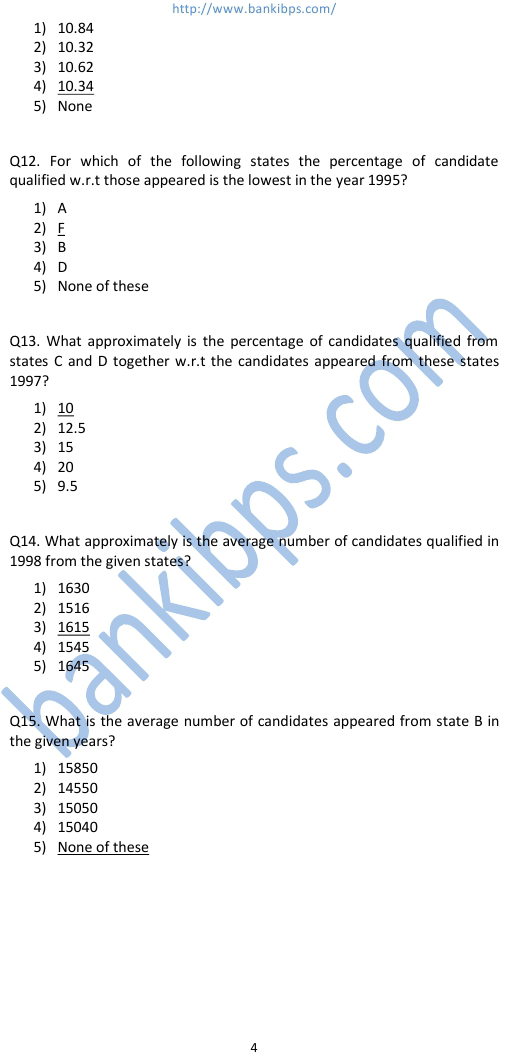# Data Analysis Sample Questions

Q1. Expenditure of company B in 1996 and 1997 are Rs 12 lakh and Rs 43.5 lakhs respectively. What was the total data analysis sample questions income of company B in 1996 and 1997 together ( in lakh rupees)? 1) 39.75 2) 37.95 3) 38.75 4) 38.5 5) None of these Q2. Ratio of expenditure of companies A and B in 1999 was 3:4. What was the ration of their income in 1999? 1) 21:26 2) 13:14 3) 14:13 4) 26:21 5) None of these Q3. Total of expenditure of companies A in all the years together was Rs 82.5 lakh. What was the total income of the company in all the years together? 1) Rs 1.23 crores 2) Rs 98.75 lakhs 3) Rs 99.85 lakhs 4) Can’t be determined 5) None of these
Practice Exercise - 232 [ Data Analysis ]## data analysis sample questions

### data analysis samples

#### sample analysis and interpretation of data

##### data and data analysis
###### data analysis tests
data analysis samples. data analysis plan sample. sample of data analysis. sample data analysis. sample of data analysis and interpretation. sample analysis and interpretation of data. data analysis practice problems. data analysis test questions. data analysis test. what is a data analysis. data and data analysis. online data analysis. data analysis tests. data analysis online.